# Distance between Mars and Earth

MMS
Hi guys,

There are many calculators and applications out there that can calculate you the distance between Earth and Mars at every time, but I'm trying to find the actual function that gives me so and draw the path of it.

My objective is finding the distance R (and Phi of t), as shown below, as a function of time.
I'm trying to sort out some geometry here but I keep getting functions that are implicit (with R and Phi).

Any ideas how to find R and Phi and as a function of time each explicitly?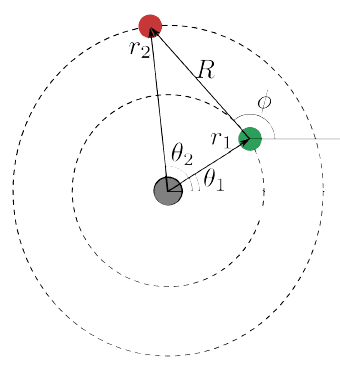Gold Member
I don't do orbital mechanics myself, but I know that it's a lot more complex than you seem to think. To start with, both orbits are elliptical, not circular, and of course their speeds are different.
Here's a starting point: http://en.wikipedia.org/wiki/Orbital_mechanics

Mentor
What is phi supposed to represent?

You can resolve the polar coordinates into cartesian coordinates, and then easily determine the distance.

Chet

MMS
I don't do orbital mechanics myself, but I know that it's a lot more complex than you seem to think. To start with, both orbits are elliptical, not circular, and of course their speeds are different.
Here's a starting point: http://en.wikipedia.org/wiki/Orbital_mechanics

Approximately speaking, they're circular. I can tell you both their time periods aswell (Kepler's 3rd law).

What is phi supposed to represent?

You can resolve the polar coordinates into cartesian coordinates, and then easily determine the distance.

Chet

It's simply the angle that is defined in the image.

Gold Member
By the way, the path that I'm trying to draw (in some program of course) is the following:
Okay, sorry. I thought that you meant something that you could use to programme a spacecraft trajectory.

Mentor
It's simply the angle that is defined in the image.
You have a line drawn from mars to earth that is also tangent to earth's orbit. I hope you realize that you can't generally do this.

Chet

MMS
You have a line drawn from mars to earth that is also tangent to earth's orbit. I hope you realize that you can't generally do this.

Chet

In that specific time it is shown to be tangent (as if someone snapped a picture at that time). Of course, it isn't the general case which I'm looking for.

MMS
Okay, sorry. I thought that you meant something that you could use to programme a spacecraft trajectory.
I'm still far from orbital mechanics so no need to worry about that now. :D

Mentor
In that specific time it is shown to be tangent (as if someone snapped a picture at that time). Of course, it isn't the general case which I'm looking for.
So, again, what does phi look like when it's not tangent, and why do you want to know phi?

MMS
So, again, what does phi look like when it's not tangent, and why do you want to know phi?

This could be phi at a different time: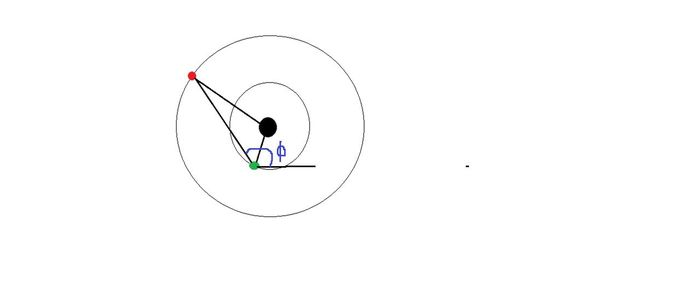Knowing phi and R as a function of time helps me plot its path as shown below.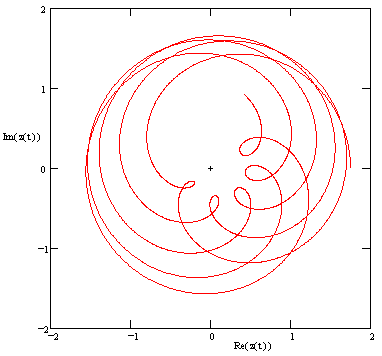MMS
I believe I have reached the answers. I'd be happy if someone could give it a look:

My final answer for R(t) using the law of cosine is: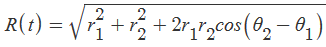And phi(t) using arctan(y/x) for R: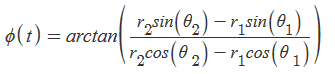Mentor
I believe I have reached the answers. I'd be happy if someone could give it a look:

My final answer for R(t) using the law of cosine is:And phi(t) using arctan(y/x) for R: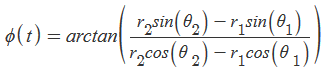Yes. That looks correct.

Chet